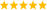LabVIEW

cancel
Showing results for
Did you mean:

Highlighted

Drawing Tangent to the Circle using a External Point

From the Following Image, The Point P(x,y), Radius(R), Center (0,0) are known values. My aim is to Find the two possible tangents which intersect the Circle at T1(x1,y1) and T2(x2,y2).

In Labview, I am not using Picture plot functions to do this. I use some mathematical formula and I showing the Output in XY Graph. Some Plotting is based on Co-ordinate points (i.e.., x and y).

I want to know the point ( T1 and T2 ) which intersect the circle as a Tangent line which is passing through the Common point P(x,y). How I can do in Labview..?Regards

Gokul

Message 1 of 4
(692 Views)

Re: Drawing Tangent to the Circle using a External Point

Well, you say you have the formula. What prevents you from just implementing it in LabVIEW?What have you tried so far and what problems did you encounter? Is this a homework question?LabVIEW ChampionDo more with less code and in less timeMessage 2 of 4
(688 Views)

Re: Drawing Tangent to the Circle using a External Point

This is not a Homework Question . Its a little part of my project which is Designing CAM Profile.. So far I didn't get any problem. Now I want to find the value that two co-ordinate points by using those given inputs. That's it...

Regards

Gokul

Message 3 of 4
(682 Views)

Re: Drawing Tangent to the Circle using a External Point

gokul2013 wrote:

This is not a Homework Question . Its a little part of my project which is Designing CAM Profile.. So far I didn't get any problem. Now I want to find the value that two co-ordinate points by using those given inputs. That's it...

So that's a math question, not a LabVIEW question. Start reading here. See how far you get...LabVIEW ChampionDo more with less code and in less timeMessage 4 of 4
(675 Views)